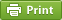Werner's Blog — Opinion, Analysis, Commentary
Bivariate Uniform Random Numbers

About a year ago I explained the math behind generating bivariate normal random numbers. This blog continues the story with a look at bivariate uniform random numbers that are correlated. It is not as straight-forward as one may think. In preparing this blog I have relied on the work in Demirtas (2014), referenced below.

The problem: you want to generate a pair of random numbers $$x$$ and $$y$$ that are drawn from the standard uniform distribution $$U\sim[0,1]$$ with a Pearson correlation coefficient $$\rho\in[-1,+1]$$. There are three trivial cases. When the two uniform random variables are uncorrelated, simply draw two uniform random numbers. When they are perfectly correlated, draw one random number $$u$$. If the two numbers are positively correlated $$\rho=+1$$, then $$x=y=u$$. If the two numbers are negatively correlated $$\rho=-1$$, then $$x=u$$ and $$y=1-u$$. But how do we get random numbers for arbitrary $$\rho$$?

To generate a pair of correlated uniform random numbers, we need to start with drawing two uncorrelated uniform random numbers—let us call them $$u$$ and $$v$$. The first draw remains untransformed so that $$x=u$$. The second draw is transformed so that it reflects the correlation structure. For this we actually need a third random draw, but from a beta distribution. Recall that a Beta distribution has two shape parameters and is also defined on the [0,1] interval just like the standard uniform distribution. Specifically, we need a draw $$w$$ from the distribution $$B(\alpha,1)=\Gamma(\alpha)/\Gamma(\alpha+1)=1/\alpha$$ with shape parameter $\alpha=\frac{1}{2}\left[\sqrt{\frac{49+\rho}{1+\rho}}-5\right]$ This distribution has mean $$\alpha/(1+\alpha)$$ with probability density function $$\alpha\cdot x^{\alpha-1}$$. The last step is to take the uniform random draw $$v$$ and the beta random draw $$w$$ and combine them to determine $$y$$: $y=\left\{\begin{array}{ll}|w-x|&\mathrm{if~}v<1/2\\ 1-|1-w-x|&\mathrm{if~}v\ge1/2\end{array}\right.$

The function $$\alpha(\rho)$$ is monotonically decreasing in $$\rho$$. It goes from $$\alpha(-1)\rightarrow\infty$$ over $$\alpha(0)=1$$ to $$\alpha(+1)=0$$. This feature means that calculations with a $$\rho$$ extremely close to negative one may become numerically unstable. But even -0.9988577955 still delivers a manageable $$\alpha=100$$.

The following R code illustrates how to create a matrix of size $$n\times2$$ with $$n$$ pairs of correlated uniform random numbers. Pass the size "n" and correlation coefficient "rho" to function rbunif to get back a the $$n\times2$$ matrix of pairs of correlated uniform random numbers.

// bivariate uniform random variables rbvunif <- function(n,rho) { x <- runif(n) if ((rho > 1.0) || (rho < -1.0)) { stop('rbvunif::rho not in [-1,+1]') } else if (rho==1.0) xy <- cbind(x,x) else if (rho==-1.0) xy <- cbind(x,1-x) else if (rho==0.0) xy <- cbind(x,runif(n)) else { a <- (sqrt((49+rho)/(1+rho))-5)/2 u <- rbeta(n,a,1.0) y <- runif(n) y <- ifelse(y<0.5 ,abs(u-x), 1-abs(1-u-x) ) xy <- cbind(x,y) } return(xy) } z <- rbvunif(5000,0.5) print(cor(z[,1],z[,2])) z <- rbvunif(5000,-0.5) print(cor(z[,1],z[,2]))

Originally I was interested in the bivariate uniform distribution function for analytic rather than numeric purposes. The cumulative density function (CDF) turns out to be a bit unwieldy because it depends on the region. The $$[0,1]^2$$ square can be divided into four regions (south, west, east, north) by putting an X across the mid point and describing each of the four triangles.

$F(x,y)=\left\{\begin{array}{ll} (G-J)/L &\quad x\le y, x\le 1-y\quad\mathrm{south}\\ (G-H)/L &\quad x\ge y, x\le 1-y\quad\mathrm{west}\\ y-1+x+(K-J)/L &\quad x\le y, x\ge 1-y\quad\mathrm{east}\\ y-1+x+(K-H)/L &\quad x\ge y, x\ge 1-y\quad\mathrm{north} \end{array}\right.$ with $\begin{array}{rl} G\equiv&(x+y)^{1+\alpha}\\ H\equiv&(x-y)^{1+\alpha}\\ J\equiv&(y-x)^{1+\alpha}\\ K\equiv&(2-x-y)^{1+\alpha}\\ L\equiv&2(1+\alpha)\end{array}$

Analytic work that tries to integrate over two correlated uniform distributions is generally not tractable. It is much easier to work with a correlated bivariate Bernoulli distribution where the outcomes are binary rather than continuous. There are some special cases where tractability can be achieved with a correlated uniform distribution. Consider the application where we are only interested in the symmetric CDF where $$x=y$$ and thus we only have two cases:

$F(x,x)=\left\{\begin{array}{ll} (2^\alpha x^{1+\alpha})/(1+\alpha) &\quad x\le 1/2\\ 2x-1+2^\alpha (1-x)^{1+\alpha}/(1+\alpha) &\quad x>1/2 \end{array}\right.$

When one is only interested in symmetric cases the above formula can be quite useful especially for specific correlations. Consider the case with negative correlation $$\rho=-0.4$$ so that $$\alpha=2$$, in which case $$F(x,x)=(4/3)x^3$$ and $$F(x,x)=2x-1+(4/3)\cdot(1-x)^3$$, respectively, with $$F(0.5,0.5)=1/6$$.

To researchers, the probability distribution of the sum of the two correlated random variables may be of particular interest. Deriving the probabilty that observations are located in th triangle $$x+y\le s$$ can be shown to follow $\mathrm{Prob}(x+y\le s)= \left\{\begin{array}{ll} s^{1+\alpha}/2&s\in[0,1)\\ 1/2&s=1\\ 1-(2-s)^{1+\alpha}&s\in(1,2] \end{array}\right.$ This derivation follows symmetry arguments, and it is clear that half the observations are always each side of the diagonal line $$x+y=1$$ regardless of the correlation. When the data are perfectly positvely correlated, the above function is perfectly linear. When the data are perfectly negatively correlated, the function is a pefect step function at $$s=1$$.

It is a pity that the correlated bivariate uniform distribution is not making it easy to use it for analytic modelling. It is always possible to numerically integrate and simulate, but the beauty of analytic work—deriving closed form solutions—is lost.

Posted on Sunday, July 5, 2020 at 07:30 — #Econometrics | #SoftwareRecent Blog Entries

Topics

Months

Subscribe to RSS feed© 2023  Prof. Werner Antweiler, University of British Columbia.